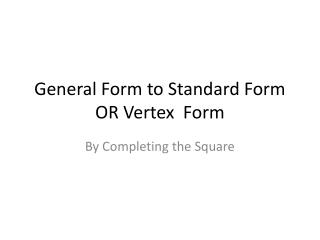DownloadDownload PresentationGeneral Form to Standard Form OR Vertex Form

# General Form to Standard Form OR Vertex Form

Download Presentation## General Form to Standard Form OR Vertex Form

- - - - - - - - - - - - - - - - - - - - - - - - - - - E N D - - - - - - - - - - - - - - - - - - - - - - - - - - -
##### Presentation Transcript

1. General Form to Standard Form OR Vertex Form By Completing the Square

2. General Form of Conics

3. + Cx + Dy + E = 0 This term complicates things… we’ll leave it for Precal. All conic sections can be written in the general form Ax2 + Bxy + Cy2 + Dx + Ey+ F = 0. All conic sections can be written in the general form Ax2 + Bxy + Cy2 + Dx + Ey+ F = 0. How many squared terms? Are and both present? Do they have the same sign? Do they havethe samecoefficients? two yes yes Circle one no Parabola no Hyperbola Ellipse

4. Circle Problem: Step 1 ---Rearrange the equation: ---x terms together on the left with a blank ---y terms together on the left with a blank ---constants on the right with 2 blanks Step 2 ---Fill in the blanks: ---half of the x term squared goes in the x blank and on the other side. ---half of the y term squared goes in the y blank and on the other side Step 3 ---factor the x trinomial ---factor the y trinomial ---Identify the conic

5. Ellipse Problem: Step 1 ---Rearrange the equation: ---x terms together on the left with a blank ---y terms together on the left with a blank constants on the right with 2 blanks Step 2 ---Fill in the blanks: ---half of the x term squared goes in the x blank and on the other side. ---half of the y term squared goes in the y blank and on the other side ---multiply them by the numbers outside for the other blanks Step 3 ---factor the x trinomial ---factor the y trinomial ---Divide both sides by the constant on the right hand side. ---Identify the conic

6. Parabola Problem: Step 1 ---non-squared variable is moved to the right along with the constant and a blank ---squared variable and corresponding terms on left with a blank Step 2 ---half the middle term squared goes in both blanks ---factor the squared trinomial ---factor out common value on the right Step 3 ---Move the factored out value to the other side by multiplying by the reciprocal. ---Identify the conic

7. Hyperbola Problem: Step 1 ---Rearrange the equation: ---x terms together on the left with a blank ---y terms together on the left with a blank ---constants on the right with 2 blanks Step 2 ---Fill in the blanks: ---half of the x term squared goes in the x blank and on the other side. ---half of the y term squared goes in the y blank and on the other side ---multiply them by the numbers outside for the other blanks Step 3 ---factor the x trinomial ---factor the y trinomial ---Divide both sides by the constant on the right hand side. ---Identify the conic

8. On Your Own a) b)

9. On Your Own c) d) • x² + 8y + 4x - 4 = 0